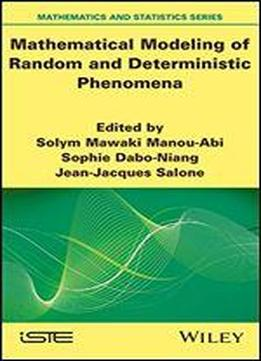# Mathematical Modeling Of Random And Deterministic Phenomena by Solym Mawaki Manou-Abi / 2020 / English / PDF

This book highlights mathematical research interests that appear in real life, such as the study and modeling of random and deterministic phenomena. As such, it provides current research in mathematics, with applications in biological and environmental sciences, ecology, epidemiology and social perspectives. The chapters can be read independently of each other, with dedicated references specific to each chapter. The book is organized in two main parts. The first is devoted to some advanced mathematical problems regarding epidemic models predictions of biomass space-time modeling of extreme rainfall modeling with the piecewise deterministic Markov process optimal control problems evolution equations in a periodic environment and the analysis of the heat equation. The second is devoted to a modelization with interdisciplinarity in ecological, socio-economic, epistemological, demographic and social problems. Mathematical Modeling of Random and Deterministic Phenomena is aimed at expert readers, young researchers, plus graduate and advanced undergraduate students who are interested in probability, statistics, modeling and mathematical analysis.

views: 374